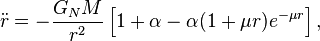# Dark Matter or New Math?Dark Matter” is the raison d’être for what holds galaxies together.

For the past couple of years, television and popular science articles have been capitalizing this popular theory. It came about because movements on a galactic scale found Newtons law of universal gravitation (1687) as modified by Albert Einstein’s General Relativity (1915) to be inadequate. According to those theories, the galaxies do not have sufficient gravity for their observed sizes. They should fly apart.

But they don’t.

Hence, the presence of some dark matter — matter than cannot be seen and does not interact with normal matter in any way except by gravity — was postulated as a solution to this problem. (So-called dark energy is similarly postulated.)

But this is not the only proposal. Others include MONDTeVeSMOG or STV gravity, and the phenomenological covariant approach.

These latter proposals are less dramatic but perhaps because of that, they receive less attention in the popular press.

We will take a brief, mostly non-mathematical, look at each of them.

MOND or Modified Newtonian dynamics explains some of the galactic observations by tweaking Newton’s formula for the law of universal gravitation. In its most basic terms, that law states that the greater an object’s mass, the stronger its gravity.

MOND, however, says that at extremely low accelerations such as those encountered by stars rotating around a galactic center (rather than proceeding in a straight line outward), Newton’s law needs an adjustment. This would take the form of an additional term that is significant only at exceedingly small accelerations.

This addition of new terms to account for exceptional situations is not uncommon in the realm of physics. Indeed, much of Einstein’s work on Special and then later his General Theory of Relativity essentially follows this practice.

Unfortunately, MOND fails to account for some of the troubling observations. It is, perhaps, a step in the right direction but is otherwise incomplete.

Next, TeVes (Tensor-Vector-Scalar gravity) starts with MOND but adds more terms. It does a better job of accounting for actual observations. Unfortunately, it also falls short. Although we seem to be headed in the right direction of changing the math to match reality rather than inventing new matter, we’re not there yet.

MOG (Modified Gravity) or STV gravity, or simply STVG (Scalar-Tensor-Vector Gravity, is the next idea and not to be confused with the Tensor-Vector-Scalar gravity theory of the previous paragraph [Phew!]). Wikipedia explains MOG by saying that, “far from a source, gravity is stronger than the Newtonian prediction, but at shorter distances, it is counteracted by a repulsive fifth force…”

Here is the modified formula for the strength of an object’s gravity:$\ddot{r}=-\frac{G_NM}{r^2}\left[1+\alpha-\alpha(1+\mu r)e^{-\mu r}\right]$

which, you may note, simply adds the bracketed expression to what is otherwise Newton’s historic expression that says the strength of an object’s gravity is its mass (M) multiplied by a constant (Gn) that then decreases with the square of the distance (“r” squared) from that object.

In other words, it is Newton’s equation with another term added, the one in the square brackets.

And while the invention of a new fifth force in addition to the traditional four — gravitationalelectromagneticstrong nuclear, and weak nuclear — may put this theory somewhat in the same category as does the invention of dark matter (and dark energy), it does so without adding something we cannot sense other than by its gravity.

Unlike the invention of dark matter (and energy), I find the discovery of a simple and subtle new force much more palatable.

Finally, the Phenomenological covariant approach follows the same general strategy by again proposing new terms to the math rather than inventing “dark” matter. The abstract states, “This modification requires introducing a single new universal parameter. It requires inclusion of neither dark matter nor dark energy.”

This theory, as do all four, adds to the math rather than proposing new matter and energy with bizarre characteristics.

But at this point I must confess that the math in all of these approaches is well beyond my ability to manipulate or fully understand.

They do, however, share the same approach of tweaking the math of the universe rather than inventing a whole new class of mass and energy. For that, I find them far more agreeable.

Personally, I’m betting on MOG if, for no other reason, than it’s the easiest to pronounce.

## 1 thought on “Dark Matter or New Math?”

1. Why the galaxy rotation curves are almost flat? To make the curves flat, dark matter exists with a proper distribution.
But it is reasonable to consider the possibility of the universal gravity equation for the two dimensional space with certain depth like a pizza dough. Stars like the Sun are swaying up and down confined by the steep gradiant of the gravity field of the surfaces of the disk. Inside of this pizza dough, the space is ordinarily three dimensional while the distance of objects is relatively short, for example, within the solar system.
For the two dimensional universal gravity equation; It can be called “New2ton’s equation”.
F can be transformed into F = G’Mm / r
Here G’ = the two dimensional universal gravity constant
F = G’Mm / r → G’Mm / r = mv2 / r
v = const = ( G’M )1/2
M ≈ M(<r) ∝ v2 = constant for each galaxy